Intermediate Algebra 2e

# 9.7Graph Quadratic Functions Using Transformations

Intermediate Algebra 2e9.7 Graph Quadratic Functions Using Transformations

### Learning Objectives

By the end of this section, you will be able to:

• • Graph quadratic equations of the form $f(x)=x2+kf(x)=x2+k$
• • Graph quadratic functions of the form $f(x)=(x-h)2f(x)=(x-h)2$
• Graph quadratic functions of the form $f(x)=ax2f(x)=ax2$
• Graph quadratic functions using transformations
• Find a quadratic function from its graph

### Be Prepared 9.19

Before you get started, take this readiness quiz.

Graph the function $f(x)=x2f(x)=x2$ by plotting points.
If you missed this problem, review Example 3.54.

### Be Prepared 9.20

Factor completely: $y2−14y+49.y2−14y+49.$
If you missed this problem, review Example 6.24.

### Be Prepared 9.21

Factor completely: $2x2−16x+32.2x2−16x+32.$
If you missed this problem, review Example 6.26.

### Graph Quadratic Functions of the form $f(x)=x2+kf(x)=x2+k$

In the last section, we learned how to graph quadratic functions using their properties. Another method involves starting with the basic graph of $f(x)=x2f(x)=x2$ and ‘moving’ it according to information given in the function equation. We call this graphing quadratic functions using transformations.

In the first example, we will graph the quadratic function $f(x)=x2f(x)=x2$ by plotting points. Then we will see what effect adding a constant, k, to the equation will have on the graph of the new function $f(x)=x2+k.f(x)=x2+k.$

### Example 9.53

Graph $f(x)=x2,g(x)=x2+2,f(x)=x2,g(x)=x2+2,$ and $h(x)=x2−2h(x)=x2−2$ on the same rectangular coordinate system. Describe what effect adding a constant to the function has on the basic parabola.

### Try It 9.105

Graph $f(x)=x2,g(x)=x2+1,f(x)=x2,g(x)=x2+1,$ and $h(x)=x2−1h(x)=x2−1$ on the same rectangular coordinate system.
Describe what effect adding a constant to the function has on the basic parabola.

### Try It 9.106

Graph $f(x)=x2,g(x)=x2+6,f(x)=x2,g(x)=x2+6,$ and $h(x)=x2−6h(x)=x2−6$ on the same rectangular coordinate system.
Describe what effect adding a constant to the function has on the basic parabola.

The last example shows us that to graph a quadratic function of the form $f(x)=x2+k,f(x)=x2+k,$ we take the basic parabola graph of $f(x)=x2f(x)=x2$ and vertically shift it up $(k>0)(k>0)$ or shift it down $(k<0)(k<0)$.

This transformation is called a vertical shift.

### Graph a Quadratic Function of the form $f ( x ) = x 2 + k f ( x ) = x 2 + k$ Using a Vertical Shift

The graph of $f(x)=x2+kf(x)=x2+k$ shifts the graph of $f(x)=x2f(x)=x2$ vertically k units.

• If k > 0, shift the parabola vertically up k units.
• If k < 0, shift the parabola vertically down $|k||k|$ units.

Now that we have seen the effect of the constant, k, it is easy to graph functions of the form $f(x)=x2+k.f(x)=x2+k.$ We just start with the basic parabola of $f(x)=x2f(x)=x2$ and then shift it up or down.

It may be helpful to practice sketching $f(x)=x2f(x)=x2$ quickly. We know the values and can sketch the graph from there.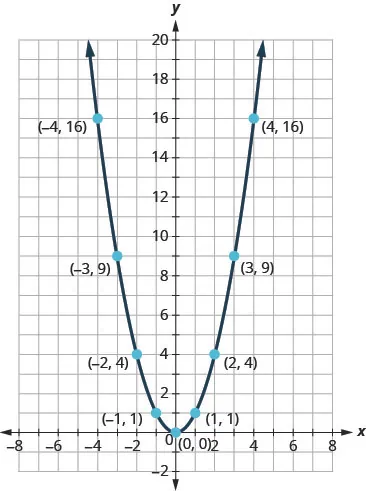Once we know this parabola, it will be easy to apply the transformations. The next example will require a vertical shift.

### Example 9.54

Graph $f(x)=x2−3f(x)=x2−3$ using a vertical shift.

### Try It 9.107

Graph $f(x)=x2−5f(x)=x2−5$ using a vertical shift.

### Try It 9.108

Graph $f(x)=x2+7f(x)=x2+7$ using a vertical shift.

### Graph Quadratic Functions of the form $f(x)=(x−h)2f(x)=(x−h)2$

In the first example, we graphed the quadratic function $f(x)=x2f(x)=x2$ by plotting points and then saw the effect of adding a constant k to the function had on the resulting graph of the new function $f(x)=x2+k.f(x)=x2+k.$

We will now explore the effect of subtracting a constant, h, from x has on the resulting graph of the new function $f(x)=(x−h)2.f(x)=(x−h)2.$

### Example 9.55

Graph $f(x)=x2,g(x)=(x−1)2,f(x)=x2,g(x)=(x−1)2,$ and $h(x)=(x+1)2h(x)=(x+1)2$ on the same rectangular coordinate system. Describe what effect adding a constant to the function has on the basic parabola.

### Try It 9.109

Graph $f(x)=x2,g(x)=(x+2)2,f(x)=x2,g(x)=(x+2)2,$ and $h(x)=(x−2)2h(x)=(x−2)2$ on the same rectangular coordinate system.
Describe what effect adding a constant to the function has on the basic parabola.

### Try It 9.110

Graph $f(x)=x2,g(x)=x2+5,f(x)=x2,g(x)=x2+5,$ and $h(x)=x2−5h(x)=x2−5$ on the same rectangular coordinate system.
Describe what effect adding a constant to the function has on the basic parabola.

The last example shows us that to graph a quadratic function of the form $f(x)=(x−h)2,f(x)=(x−h)2,$ we take the basic parabola graph of $f(x)=x2f(x)=x2$ and shift it left (h > 0) or shift it right (h < 0).

This transformation is called a horizontal shift.

### Graph a Quadratic Function of the form $f ( x ) = ( x − h ) 2 f ( x ) = ( x − h ) 2$ Using a Horizontal Shift

The graph of $f(x)=(x−h)2f(x)=(x−h)2$ shifts the graph of $f(x)=x2f(x)=x2$ horizontally $hh$ units.

• If h > 0, shift the parabola horizontally right h units.
• If h < 0, shift the parabola horizontally left $|h||h|$ units.

Now that we have seen the effect of the constant, h, it is easy to graph functions of the form $f(x)=(x−h)2.f(x)=(x−h)2.$ We just start with the basic parabola of $f(x)=x2f(x)=x2$ and then shift it left or right.

The next example will require a horizontal shift.

### Example 9.56

Graph $f(x)=(x−6)2f(x)=(x−6)2$ using a horizontal shift.

### Try It 9.111

Graph $f(x)=(x−4)2f(x)=(x−4)2$ using a horizontal shift.

### Try It 9.112

Graph $f(x)=(x+6)2f(x)=(x+6)2$ using a horizontal shift.

Now that we know the effect of the constants h and k, we will graph a quadratic function of the form $f(x)=(x−h)2+kf(x)=(x−h)2+k$ by first drawing the basic parabola and then making a horizontal shift followed by a vertical shift. We could do the vertical shift followed by the horizontal shift, but most students prefer the horizontal shift followed by the vertical.

### Example 9.57

Graph $f(x)=(x+1)2−2f(x)=(x+1)2−2$ using transformations.

### Try It 9.113

Graph $f(x)=(x+2)2−3f(x)=(x+2)2−3$ using transformations.

### Try It 9.114

Graph $f(x)=(x−3)2+1f(x)=(x−3)2+1$ using transformations.

### Graph Quadratic Functions of the Form $f(x)=ax2f(x)=ax2$

So far we graphed the quadratic function $f(x)=x2f(x)=x2$ and then saw the effect of including a constant h or k in the equation had on the resulting graph of the new function. We will now explore the effect of the coefficient a on the resulting graph of the new function $f(x)=ax2.f(x)=ax2.$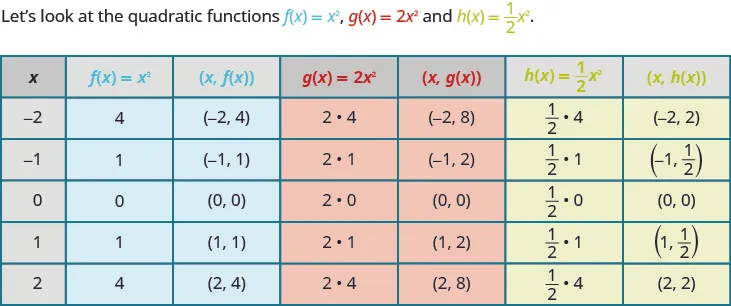If we graph these functions, we can see the effect of the constant a, assuming a > 0.To graph a function with constant a it is easiest to choose a few points on $f(x)=x2f(x)=x2$ and multiply the y-values by a.

### Graph of a Quadratic Function of the form $f ( x ) = a x 2 f ( x ) = a x 2$

The coefficient a in the function $f(x)=ax2f(x)=ax2$ affects the graph of $f(x)=x2f(x)=x2$ by stretching or compressing it.

• If $0<|a|<1,0<|a|<1,$ the graph of $f(x)=ax2f(x)=ax2$ will be “wider” than the graph of $f(x)=x2.f(x)=x2.$
• If $|a|>1|a|>1$, the graph of $f(x)=ax2f(x)=ax2$ will be “skinnier” than the graph of $f(x)=x2.f(x)=x2.$

### Example 9.58

Graph $f(x)=3x2.f(x)=3x2.$

### Try It 9.115

Graph $f(x)=−3x2.f(x)=−3x2.$

### Try It 9.116

Graph $f(x)=2x2.f(x)=2x2.$

### Graph Quadratic Functions Using Transformations

We have learned how the constants a, h, and k in the functions, $f(x)=x2+k,f(x)=(x−h)2,f(x)=x2+k,f(x)=(x−h)2,$ and $f(x)=ax2f(x)=ax2$ affect their graphs. We can now put this together and graph quadratic functions $f(x)=ax2+bx+cf(x)=ax2+bx+c$ by first putting them into the form $f(x)=a(x−h)2+kf(x)=a(x−h)2+k$ by completing the square. This form is sometimes known as the vertex form or standard form.

We must be careful to both add and subtract the number to the SAME side of the function to complete the square. We cannot add the number to both sides as we did when we completed the square with quadratic equations.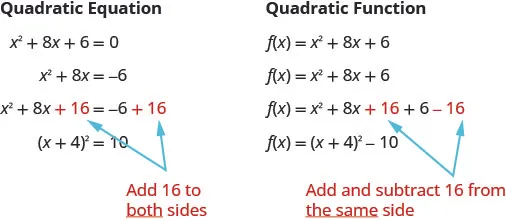When we complete the square in a function with a coefficient of x2 that is not one, we have to factor that coefficient from just the x-terms. We do not factor it from the constant term. It is often helpful to move the constant term a bit to the right to make it easier to focus only on the x-terms.

Once we get the constant we want to complete the square, we must remember to multiply it by that coefficient before we then subtract it.

### Example 9.59

Rewrite $f(x)=−3x2−6x−1f(x)=−3x2−6x−1$ in the $f(x)=a(x−h)2+kf(x)=a(x−h)2+k$ form by completing the square.

### Try It 9.117

Rewrite $f(x)=−4x2−8x+1f(x)=−4x2−8x+1$ in the $f(x)=a(x−h)2+kf(x)=a(x−h)2+k$ form by completing the square.

### Try It 9.118

Rewrite $f(x)=2x2−8x+3f(x)=2x2−8x+3$ in the $f(x)=a(x−h)2+kf(x)=a(x−h)2+k$ form by completing the square.

Once we put the function into the $f(x)=(x−h)2+kf(x)=(x−h)2+k$ form, we can then use the transformations as we did in the last few problems. The next example will show us how to do this.

### Example 9.60

Graph $f(x)=x2+6x+5f(x)=x2+6x+5$ by using transformations.

### Try It 9.119

Graph $f(x)=x2+2x−3f(x)=x2+2x−3$ by using transformations.

### Try It 9.120

Graph $f(x)=x2−8x+12f(x)=x2−8x+12$ by using transformations.

We list the steps to take to graph a quadratic function using transformations here.

### How To

#### Graph a quadratic function using transformations.

1. Step 1. Rewrite the function in $f(x)=a(x−h)2+kf(x)=a(x−h)2+k$ form by completing the square.
2. Step 2. Graph the function using transformations.

### Example 9.61

Graph $f(x)=−2x2−4x+2f(x)=−2x2−4x+2$ by using transformations.

### Try It 9.121

Graph $f(x)=−3x2+12x−4f(x)=−3x2+12x−4$ by using transformations.

### Try It 9.122

Graph $f(x)=−2x2+12x−9f(x)=−2x2+12x−9$ by using transformations.

Now that we have completed the square to put a quadratic function into $f(x)=a(x−h)2+kf(x)=a(x−h)2+k$ form, we can also use this technique to graph the function using its properties as in the previous section.

If we look back at the last few examples, we see that the vertex is related to the constants h and k.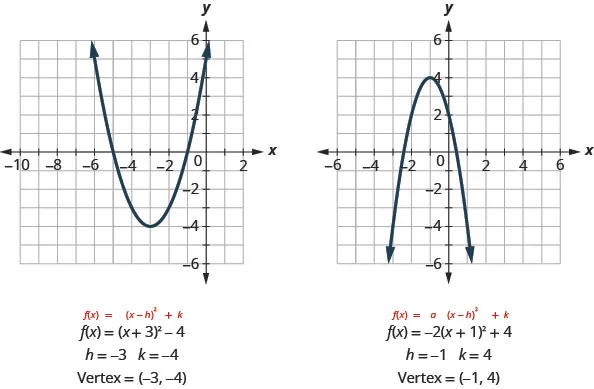In each case, the vertex is (h, k). Also the axis of symmetry is the line x = h.

We rewrite our steps for graphing a quadratic function using properties for when the function is in $f(x)=a(x−h)2+kf(x)=a(x−h)2+k$ form.

### How To

#### Graph a quadratic function in the form $f(x)=a(x−h)2+kf(x)=a(x−h)2+k$ using properties.

1. Step 1. Rewrite the function in $f(x)=a(x−h)2+kf(x)=a(x−h)2+k$ form.
2. Step 2. Determine whether the parabola opens upward, a > 0, or downward, a < 0.
3. Step 3. Find the axis of symmetry, x = h.
4. Step 4. Find the vertex, (h, k).
5. Step 5. Find the y-intercept. Find the point symmetric to the y-intercept across the axis of symmetry.
6. Step 6. Find the x-intercepts.
7. Step 7. Graph the parabola.

### Example 9.62

Rewrite $f(x)=2x2+4x+5f(x)=2x2+4x+5$ in $f(x)=a(x−h)2+kf(x)=a(x−h)2+k$ form and graph the function using properties.

### Try It 9.123

Rewrite $f(x)=3x2−6x+5f(x)=3x2−6x+5$ in $f(x)=a(x−h)2+kf(x)=a(x−h)2+k$ form and graph the function using properties.

### Try It 9.124

Rewrite $f(x)=−2x2+8x−7f(x)=−2x2+8x−7$ in $f(x)=a(x−h)2+kf(x)=a(x−h)2+k$ form and graph the function using properties.

### Find a Quadratic Function from its Graph

So far we have started with a function and then found its graph.

Now we are going to reverse the process. Starting with the graph, we will find the function.

### Example 9.63

Determine the quadratic function whose graph is shown.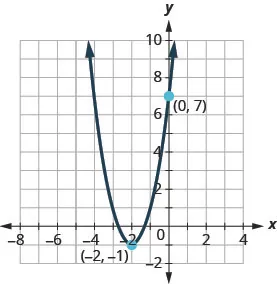### Try It 9.125

Write the quadratic function in $f(x)=a(x−h)2+kf(x)=a(x−h)2+k$ form whose graph is shown.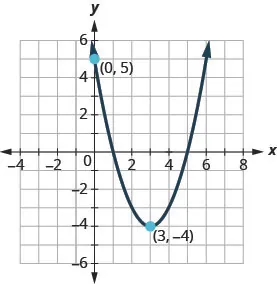### Try It 9.126

Determine the quadratic function whose graph is shown.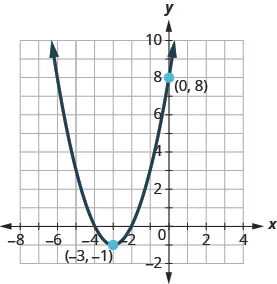### Media

Access these online resources for additional instruction and practice with graphing quadratic functions using transformations.

### Section 9.7 Exercises

#### Practice Makes Perfect

Graph Quadratic Functions of the form $f(x)=x2+kf(x)=x2+k$

In the following exercises, graph the quadratic functions on the same rectangular coordinate system and describe what effect adding a constant, k, to the function has on the basic parabola.

293.

$f(x)=x2,g(x)=x2+4,f(x)=x2,g(x)=x2+4,$ and $h(x)=x2−4.h(x)=x2−4.$

294.

$f(x)=x2,g(x)=x2+7,f(x)=x2,g(x)=x2+7,$ and $h(x)=x2−7.h(x)=x2−7.$

In the following exercises, graph each function using a vertical shift.

295.

$f ( x ) = x 2 + 3 f ( x ) = x 2 + 3$

296.

$f ( x ) = x 2 − 7 f ( x ) = x 2 − 7$

297.

$g ( x ) = x 2 + 2 g ( x ) = x 2 + 2$

298.

$g ( x ) = x 2 + 5 g ( x ) = x 2 + 5$

299.

$h ( x ) = x 2 − 4 h ( x ) = x 2 − 4$

300.

$h ( x ) = x 2 − 5 h ( x ) = x 2 − 5$

Graph Quadratic Functions of the form $f(x)=(x−h)2f(x)=(x−h)2$

In the following exercises, graph the quadratic functions on the same rectangular coordinate system and describe what effect adding a constant, $hh$, inside the parentheses has

301.

$f(x)=x2,g(x)=(x−3)2,f(x)=x2,g(x)=(x−3)2,$ and $h(x)=(x+3)2.h(x)=(x+3)2.$

302.

$f(x)=x2,g(x)=(x+4)2,f(x)=x2,g(x)=(x+4)2,$ and $h(x)=(x−4)2.h(x)=(x−4)2.$

In the following exercises, graph each function using a horizontal shift.

303.

$f ( x ) = ( x − 2 ) 2 f ( x ) = ( x − 2 ) 2$

304.

$f ( x ) = ( x − 1 ) 2 f ( x ) = ( x − 1 ) 2$

305.

$f ( x ) = ( x + 5 ) 2 f ( x ) = ( x + 5 ) 2$

306.

$f ( x ) = ( x + 3 ) 2 f ( x ) = ( x + 3 ) 2$

307.

$f ( x ) = ( x − 5 ) 2 f ( x ) = ( x − 5 ) 2$

308.

$f ( x ) = ( x + 2 ) 2 f ( x ) = ( x + 2 ) 2$

In the following exercises, graph each function using transformations.

309.

$f ( x ) = ( x + 2 ) 2 + 1 f ( x ) = ( x + 2 ) 2 + 1$

310.

$f ( x ) = ( x + 4 ) 2 + 2 f ( x ) = ( x + 4 ) 2 + 2$

311.

$f ( x ) = ( x − 1 ) 2 + 5 f ( x ) = ( x − 1 ) 2 + 5$

312.

$f ( x ) = ( x − 3 ) 2 + 4 f ( x ) = ( x − 3 ) 2 + 4$

313.

$f ( x ) = ( x + 3 ) 2 − 1 f ( x ) = ( x + 3 ) 2 − 1$

314.

$f ( x ) = ( x + 5 ) 2 − 2 f ( x ) = ( x + 5 ) 2 − 2$

315.

$f ( x ) = ( x − 4 ) 2 − 3 f ( x ) = ( x − 4 ) 2 − 3$

316.

$f ( x ) = ( x − 6 ) 2 − 2 f ( x ) = ( x − 6 ) 2 − 2$

Graph Quadratic Functions of the form $f(x)=ax2f(x)=ax2$

In the following exercises, graph each function.

317.

$f ( x ) = −2 x 2 f ( x ) = −2 x 2$

318.

$f ( x ) = 4 x 2 f ( x ) = 4 x 2$

319.

$f ( x ) = −4 x 2 f ( x ) = −4 x 2$

320.

$f ( x ) = − x 2 f ( x ) = − x 2$

321.

$f ( x ) = 1 2 x 2 f ( x ) = 1 2 x 2$

322.

$f ( x ) = 1 3 x 2 f ( x ) = 1 3 x 2$

323.

$f ( x ) = 1 4 x 2 f ( x ) = 1 4 x 2$

324.

$f ( x ) = − 1 2 x 2 f ( x ) = − 1 2 x 2$

In the following exercises, rewrite each function in the $f(x)=a(x−h)2+kf(x)=a(x−h)2+k$ form by completing the square.

325.

$f ( x ) = −3 x 2 − 12 x − 5 f ( x ) = −3 x 2 − 12 x − 5$

326.

$f ( x ) = 2 x 2 − 12 x + 7 f ( x ) = 2 x 2 − 12 x + 7$

327.

$f ( x ) = 3 x 2 + 6 x − 1 f ( x ) = 3 x 2 + 6 x − 1$

328.

$f ( x ) = −4 x 2 − 16 x − 9 f ( x ) = −4 x 2 − 16 x − 9$

In the following exercises, rewrite each function in $f(x)=a(x−h)2+kf(x)=a(x−h)2+k$ form and graph it by using transformations.

329.

$f ( x ) = x 2 + 6 x + 5 f ( x ) = x 2 + 6 x + 5$

330.

$f ( x ) = x 2 + 4 x − 12 f ( x ) = x 2 + 4 x − 12$

331.

$f ( x ) = x 2 + 4 x + 3 f ( x ) = x 2 + 4 x + 3$

332.

$f ( x ) = x 2 − 6 x + 8 f ( x ) = x 2 − 6 x + 8$

333.

$f ( x ) = x 2 − 6 x + 15 f ( x ) = x 2 − 6 x + 15$

334.

$f ( x ) = x 2 + 8 x + 10 f ( x ) = x 2 + 8 x + 10$

335.

$f ( x ) = − x 2 + 8 x − 16 f ( x ) = − x 2 + 8 x − 16$

336.

$f ( x ) = − x 2 + 2 x − 7 f ( x ) = − x 2 + 2 x − 7$

337.

$f ( x ) = − x 2 − 4 x + 2 f ( x ) = − x 2 − 4 x + 2$

338.

$f ( x ) = − x 2 + 4 x − 5 f ( x ) = − x 2 + 4 x − 5$

339.

$f ( x ) = 5 x 2 − 10 x + 8 f ( x ) = 5 x 2 − 10 x + 8$

340.

$f ( x ) = 3 x 2 + 18 x + 20 f ( x ) = 3 x 2 + 18 x + 20$

341.

$f ( x ) = 2 x 2 − 4 x + 1 f ( x ) = 2 x 2 − 4 x + 1$

342.

$f ( x ) = 3 x 2 − 6 x − 1 f ( x ) = 3 x 2 − 6 x − 1$

343.

$f ( x ) = −2 x 2 + 8 x − 10 f ( x ) = −2 x 2 + 8 x − 10$

344.

$f ( x ) = −3 x 2 + 6 x + 1 f ( x ) = −3 x 2 + 6 x + 1$

In the following exercises, rewrite each function in $f(x)=a(x−h)2+kf(x)=a(x−h)2+k$ form and graph it using properties.

345.

$f ( x ) = 2 x 2 + 4 x + 6 f ( x ) = 2 x 2 + 4 x + 6$

346.

$f ( x ) = 3 x 2 − 12 x + 7 f ( x ) = 3 x 2 − 12 x + 7$

347.

$f ( x ) = − x 2 + 2 x − 4 f ( x ) = − x 2 + 2 x − 4$

348.

$f ( x ) = −2 x 2 − 4 x − 5 f ( x ) = −2 x 2 − 4 x − 5$

Matching

In the following exercises, match the graphs to one of the following functions: $f(x)=x2+4f(x)=x2+4$ $f(x)=x2−4f(x)=x2−4$ $f(x)=(x+4)2f(x)=(x+4)2$ $f(x)=(x−4)2f(x)=(x−4)2$ $f(x)=(x+4)2−4f(x)=(x+4)2−4$ $f(x)=(x+4)2+4f(x)=(x+4)2+4$ $f(x)=(x−4)2−4f(x)=(x−4)2−4$ $f(x)=(x−4)2+4f(x)=(x−4)2+4$

349.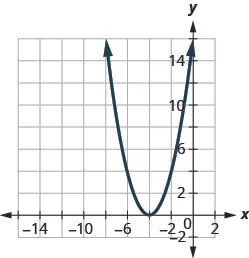350.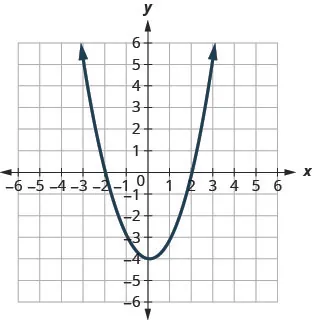351.352.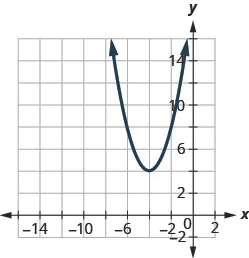353.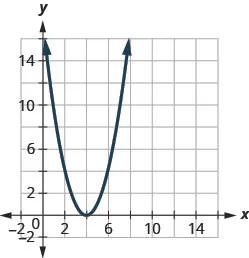354.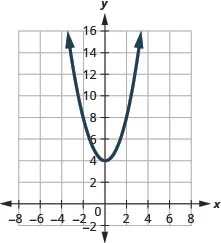355.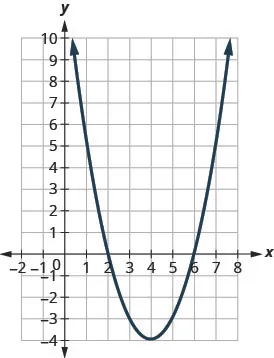356.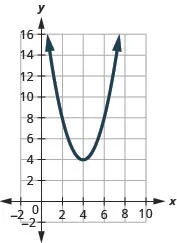Find a Quadratic Function from its Graph

In the following exercises, write the quadratic function in $f(x)=a(x−h)2+kf(x)=a(x−h)2+k$ form whose graph is shown.

357.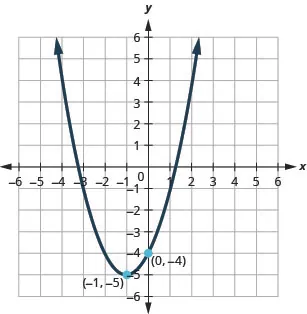358.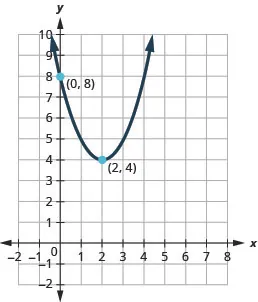359.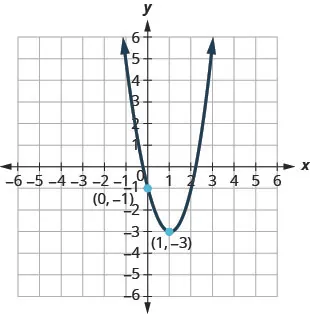360.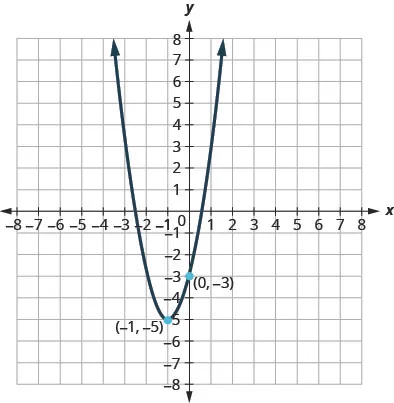#### Writing Exercise

361.

Graph the quadratic function $f(x)=x2+4x+5f(x)=x2+4x+5$ first using the properties as we did in the last section and then graph it using transformations. Which method do you prefer? Why?

362.

Graph the quadratic function $f(x)=2x2−4x−3f(x)=2x2−4x−3$ first using the properties as we did in the last section and then graph it using transformations. Which method do you prefer? Why?

#### Self Check

After completing the exercises, use this checklist to evaluate your mastery of the objectives of this section.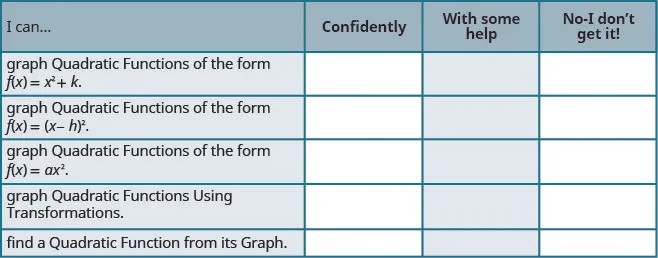After looking at the checklist, do you think you are well-prepared for the next section? Why or why not?

Order a print copy

As an Amazon Associate we earn from qualifying purchases.